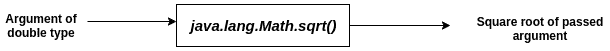# Java Math sqrt() Function: The Complete Guide

The Math.sqrt() returns the square root of a value of type double passed to it as an argument. Although the square root of any number yields both a positive and a negative result, this method only returns the positive value, leaving it to the programmer to use it accordingly.

## Java Math sqrt()

Java.lang.Math.sqrt() is a built-in method used to calculate the positive square root of any number (passed as double type). If the argument is NaN or negative, then the result is NaN. If the argument is positive infinity, then a result is positive infinity.

### Syntax

```public static double sqrt(double x)
```

### Parameter(s)

The variable of a double type whose square root is to be calculated.

### Return Value

The positive square root of the value passed.

See the following figure.#### Note

1. If the argument is NaN or negative, then this method returns NaN.
2. If an argument is a negative infinity, the sqrt() method returns NaN.
3. If an argument is positive infinity, then this method returns positive infinity.
4. If an argument is positive zero or negative zero, this method returns the same value passed.

Consider the following examples.

#### Example1.java: The following example demonstrates the use of this method.

```public class Example1 {
public static void main(String[] args) {
double num = 25.0;
System.out.println(Math.sqrt(num));
}
}
```

#### Output

```->javac Example1.java
->java Example1
5.0
```

#### Example2.java: The following example demonstrates the situation when the argument is negative or NaN.

```public class Example2 {
public static void main(String[] args) {
double num = -25.0;
System.out.println(Math.sqrt(num));
System.out.println(Math.sqrt(2.0 % 0));
}
}
```

#### Output

```->javac Example2.java
->java Example2
NaN
NaN
```

#### Example3.java: The following example demonstrates the situation of passing positive infinity.

```public class Example3 {
public static void main(String[] args) {
System.out.println(Math.sqrt(Double.POSITIVE_INFINITY));
}
}```

#### Output

```->javac Example3.java
->java Example3
Infinity
```

#### Example4.java: The following example demonstrates the situation of passing negative infinity.

```public class Example4 {
public static void main(String[] args) {
System.out.println(Math.sqrt(Double.NEGATIVE_INFINITY));
}
}
```

#### Output

```->javac Example4.java
->java Example4
NaN
```

#### Example5.java: The following example demonstrates the situation of passing negative or positive zero.

```public class Example5 {
public static void main(String[] args) {
System.out.println(Math.sqrt(0.0));
System.out.println(Math.sqrt(-0.0));
}
}
```

#### Output

```->javac Example5.java
->java Example5
0.0
-0.0
```